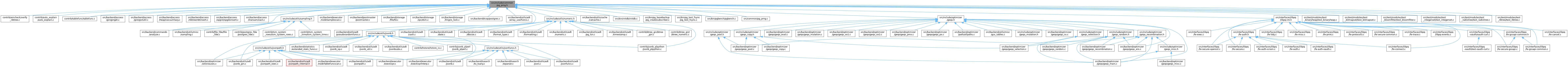PostgreSQL Source Code  git master
pg_prng.h File Reference
This graph shows which files directly or indirectly include this file:Go to the source code of this file.

## Data Structures

struct  pg_prng_state

## Macros

#define pg_prng_strong_seed(state)

## Typedefs

typedef struct pg_prng_state pg_prng_state

## Functions

void pg_prng_seed (pg_prng_state *state, uint64 seed)

void pg_prng_fseed (pg_prng_state *state, double fseed)

bool pg_prng_seed_check (pg_prng_state *state)

uint64 pg_prng_uint64 (pg_prng_state *state)

uint64 pg_prng_uint64_range (pg_prng_state *state, uint64 rmin, uint64 rmax)

int64 pg_prng_int64 (pg_prng_state *state)

int64 pg_prng_int64p (pg_prng_state *state)

uint32 pg_prng_uint32 (pg_prng_state *state)

int32 pg_prng_int32 (pg_prng_state *state)

int32 pg_prng_int32p (pg_prng_state *state)

double pg_prng_double (pg_prng_state *state)

double pg_prng_double_normal (pg_prng_state *state)

bool pg_prng_bool (pg_prng_state *state)

## Variables

PGDLLIMPORT pg_prng_state pg_global_prng_state

## ◆ pg_prng_strong_seed

 #define pg_prng_strong_seed ( state )
Value:
(pg_strong_random((void *) (state), sizeof(pg_prng_state)) ? \
pg_prng_seed_check(state) : false)
return false
Definition: isn.c:131
bool pg_strong_random(void *buf, size_t len)
Definition: regguts.h:323

Definition at line 46 of file pg_prng.h.

## ◆ pg_prng_state

 typedef struct pg_prng_state pg_prng_state

## ◆ pg_prng_bool()

 bool pg_prng_bool ( pg_prng_state * state )

Definition at line 277 of file pg_prng.c.

278 {
279  uint64 v = xoroshiro128ss(state);
280
281  return (bool) (v >> 63);
282 }
static uint64 xoroshiro128ss(pg_prng_state *state)
Definition: pg_prng.c:54

References xoroshiro128ss().

Referenced by gistchoose().

## ◆ pg_prng_double()

 double pg_prng_double ( pg_prng_state * state )

Definition at line 232 of file pg_prng.c.

233 {
234  uint64 v = xoroshiro128ss(state);
235
236  /*
237  * As above, assume there's 52 mantissa bits in a double. This result
238  * could round to 1.0 if double's precision is less than that; but we
239  * assume IEEE float arithmetic elsewhere in Postgres, so this seems OK.
240  */
241  return ldexp((double) (v >> (64 - 52)), -52);
242 }

References xoroshiro128ss().

## ◆ pg_prng_double_normal()

 double pg_prng_double_normal ( pg_prng_state * state )

Definition at line 254 of file pg_prng.c.

255 {
256  double u1,
257  u2,
258  z0;
259
260  /*
261  * pg_prng_double generates [0, 1), but for the basic version of the
262  * Box-Muller transform the two uniformly distributed random numbers are
263  * expected to be in (0, 1]; in particular we'd better not compute log(0).
264  */
265  u1 = 1.0 - pg_prng_double(state);
266  u2 = 1.0 - pg_prng_double(state);
267
268  /* Apply Box-Muller transform to get one normal-valued output */
269  z0 = sqrt(-2.0 * log(u1)) * sin(2.0 * M_PI * u2);
270  return z0;
271 }
double pg_prng_double(pg_prng_state *state)
Definition: pg_prng.c:232
#define M_PI
Definition: pg_prng.c:29

References M_PI, and pg_prng_double().

Referenced by drandom_normal(), and getGaussianRand().

## ◆ pg_prng_fseed()

 void pg_prng_fseed ( pg_prng_state * state, double fseed )

Definition at line 102 of file pg_prng.c.

103 {
104  /* Assume there's about 52 mantissa bits; the sign contributes too. */
105  int64 seed = ((double) ((UINT64CONST(1) << 52) - 1)) * fseed;
106
107  pg_prng_seed(state, (uint64) seed);
108 }
void pg_prng_seed(pg_prng_state *state, uint64 seed)
Definition: pg_prng.c:89

References pg_prng_seed().

Referenced by geqo_set_seed(), and setseed().

## ◆ pg_prng_int32()

 int32 pg_prng_int32 ( pg_prng_state * state )

Definition at line 207 of file pg_prng.c.

208 {
209  uint64 v = xoroshiro128ss(state);
210
211  return (int32) (v >> 32);
212 }
signed int int32
Definition: c.h:483

References xoroshiro128ss().

Referenced by prepare_buf().

## ◆ pg_prng_int32p()

 int32 pg_prng_int32p ( pg_prng_state * state )

Definition at line 218 of file pg_prng.c.

219 {
220  uint64 v = xoroshiro128ss(state);
221
222  return (int32) (v >> 33);
223 }

References xoroshiro128ss().

Referenced by create_and_test_bloom().

## ◆ pg_prng_int64()

 int64 pg_prng_int64 ( pg_prng_state * state )

Definition at line 173 of file pg_prng.c.

174 {
175  return (int64) xoroshiro128ss(state);
176 }

References xoroshiro128ss().

## ◆ pg_prng_int64p()

 int64 pg_prng_int64p ( pg_prng_state * state )

Definition at line 182 of file pg_prng.c.

183 {
184  return (int64) (xoroshiro128ss(state) & UINT64CONST(0x7FFFFFFFFFFFFFFF));
185 }

References xoroshiro128ss().

## ◆ pg_prng_seed()

 void pg_prng_seed ( pg_prng_state * state, uint64 seed )

Definition at line 89 of file pg_prng.c.

90 {
91  state->s0 = splitmix64(&seed);
92  state->s1 = splitmix64(&seed);
93  /* Let's just make sure we didn't get all-zeroes */
94  (void) pg_prng_seed_check(state);
95 }
static uint64 splitmix64(uint64 *state)
Definition: pg_prng.c:72
bool pg_prng_seed_check(pg_prng_state *state)
Definition: pg_prng.c:114

References pg_prng_seed_check(), and splitmix64().

## ◆ pg_prng_seed_check()

 bool pg_prng_seed_check ( pg_prng_state * state )

Definition at line 114 of file pg_prng.c.

115 {
116  /*
117  * If the seeding mechanism chanced to produce all-zeroes, insert
118  * something nonzero. Anything would do; use Knuth's LCG parameters.
119  */
120  if (unlikely(state->s0 == 0 && state->s1 == 0))
121  {
122  state->s0 = UINT64CONST(0x5851F42D4C957F2D);
123  state->s1 = UINT64CONST(0x14057B7EF767814F);
124  }
125
126  /* As a convenience for the pg_prng_strong_seed macro, return true */
127  return true;
128 }
#define unlikely(x)
Definition: c.h:300

References unlikely.

Referenced by pg_prng_seed().

## ◆ pg_prng_uint32()

 uint32 pg_prng_uint32 ( pg_prng_state * state )

Definition at line 191 of file pg_prng.c.

192 {
193  /*
194  * Although xoroshiro128** is not known to have any weaknesses in
195  * randomness of low-order bits, we prefer to use the upper bits of its
196  * result here and below.
197  */
198  uint64 v = xoroshiro128ss(state);
199
200  return (uint32) (v >> 32);
201 }
unsigned int uint32
Definition: c.h:495

References xoroshiro128ss().

## ◆ pg_prng_uint64()

 uint64 pg_prng_uint64 ( pg_prng_state * state )

Definition at line 134 of file pg_prng.c.

135 {
136  return xoroshiro128ss(state);
137 }

References xoroshiro128ss().

Referenced by bt_check_every_level(), initRandomState(), main(), and permute().

## ◆ pg_prng_uint64_range()

 uint64 pg_prng_uint64_range ( pg_prng_state * state, uint64 rmin, uint64 rmax )

Definition at line 144 of file pg_prng.c.

145 {
146  uint64 val;
147
148  if (likely(rmax > rmin))
149  {
150  /*
151  * Use bitmask rejection method to generate an offset in 0..range.
152  * Each generated val is less than twice "range", so on average we
153  * should not have to iterate more than twice.
154  */
155  uint64 range = rmax - rmin;
156  uint32 rshift = 63 - pg_leftmost_one_pos64(range);
157
158  do
159  {
160  val = xoroshiro128ss(state) >> rshift;
161  } while (val > range);
162  }
163  else
164  val = 0;
165
166  return rmin + val;
167 }
#define likely(x)
Definition: c.h:299
long val
Definition: informix.c:664
static int pg_leftmost_one_pos64(uint64 word)
Definition: pg_bitutils.h:72
static struct cvec * range(struct vars *v, chr a, chr b, int cases)
Definition: regc_locale.c:412

References likely, pg_leftmost_one_pos64(), range(), val, and xoroshiro128ss().

## ◆ pg_global_prng_state

 PGDLLIMPORT pg_prng_state pg_global_prng_state
extern